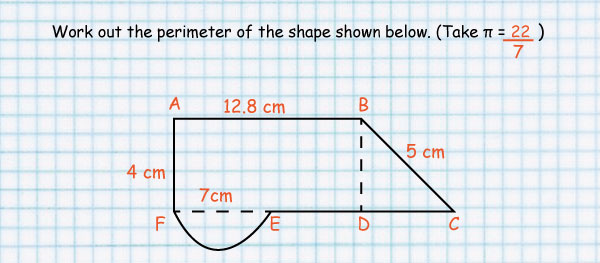Perimeter

#### Perimeter and Circumference

The distance around a shape is called its perimeter.

The perimeter of a circle is its circumference.

## How to get the Perimeter of plain shapes

#### 1. Rectangle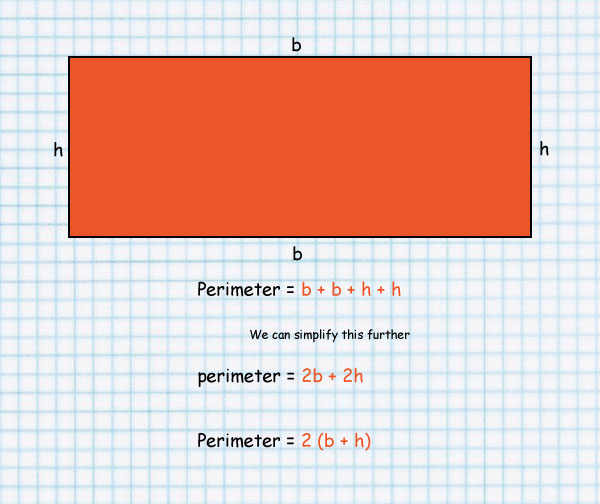#### 2. Square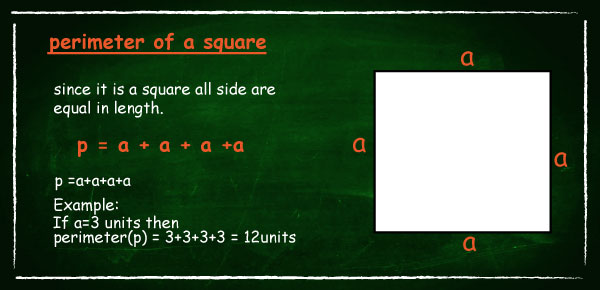#### 3. Full circle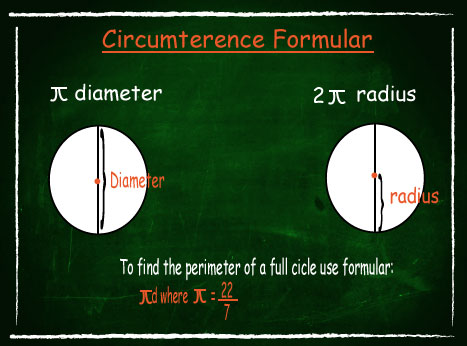####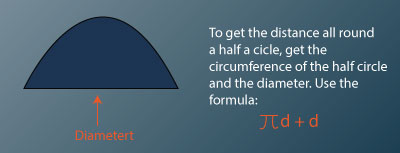• To find the perimeter of a plain shape like a rectangle, use the formula 2(L + W)
• To find the perimeter of a square use 4 X L
• To get the circumference of a full circle use πd
• To get the distance all round a half a circle, get the circumference of the half circle and the diameter. Use the formula πd + d
• To get the distance all round a figure made up of various shapes then add up the measurements.